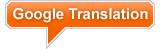Gallery Section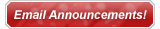### Polarized Light

Light of a single color can be described as a wave with a specified wavelength or as a photon with a specified energy. Another aspect of light is that it can be polarized with the wave vibrations lying in one plane. Many materials respond differently in their ability to transmit light depending on the relation of the plane of polarization to the crystal axes of the materials. In optical microscopy, the light beams can be polarized by use of filters.

Light is emitted from an atom when an electron makes a transition from a higher energy state to a lower energy state (Fig. 1).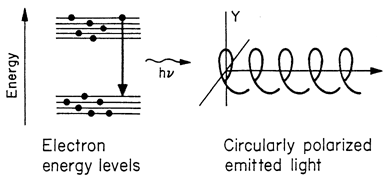Figure 1. Light (photon) emission due to an electron making a transition from a higher to a lower energy state. The emitted light carries one unit of angular momentum. The sketch at the right hand side shows circularly polarized light.

Conservation of energy applies so that the energy of light equals the energy difference between the two states. An additional constraint is that angular momentum must be conserved. The emitted photon carries away one unit of angular momentum, shown schematically on the right hand side of Fig. 1 as a rotating wave of circularly polarized light. Consequently the energy levels in the electron transition leading to light emission must differ by one unit of angular momentum. The requirement of conservation of angular momentum in light emission applies to all photons: infrared, visible light, x-rays and gamma rays.

Light can be linearly polarized as shown in Fig. 2; the light wave lies on a plane. Fig. 2 shows a horizontal and a vertical plane depiction of two linearly polarized light waves. The arrows on the right side are a conventional notation to indicate the orientation of the plane of polarization and indicate the direction of the electric vector oscillation. An unpolarized beam of light can be represented by two vector components of wave oscillations oriented at right angles to each other.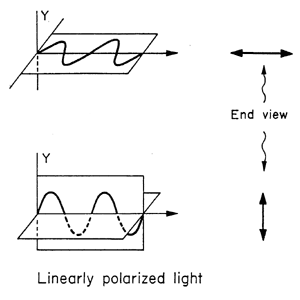Figure 2. Two examples of linearly polarized light where the electric vector of the light wave vibrates in one plane.

Human vision does not distinguish between polarized and unpolarized light. However some materials such as Polaroid can absorb certain orientations of polarized light.

Polaroid represents a class of materials that absorbs light oscillations in one direction but not the component oriented at right angles. These polarizing materials often contain long particles, rods or plates, aligned parallel to each other in a regular arrangement. These aligned particles transmit one plane of polarized light and absorb the perpendicular one as illustrated in Fig. 3. The polarizer can transform circularly polarized light into linearly polarized light.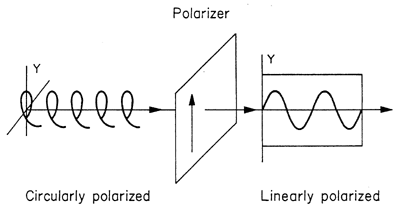Figure 3. Circularly polarized light incident on a polarizer is transmitted as linearly polarized light.

If two polarizers have their orientation axes aligned parallel to each other, the first will absorb half the unpolarized light and transmit linearly polarized light which is then transmitted without absorption through the second polarizer. The second polarizer is referred to as an "analyzer". If the analyzer is rotated by 90° so that the two polarizers are crossed with their axes perpendicular, the second polarizer will absorb all the light transmitted by the first.

Polarized light can be produced by transmission through polarizers or by reflection from the surface of transparent material such as glass or water. Fig. 4 shows incident light represented by two crossed, wave-oscillation vectors. The reflected light is polarized in the plane of the reflecting surface. The refracted beam will contain a mixture of the two orientations. If the incident light is incident at the Brewster angle, θB, the reflected light is fully polarized as shown in Fig. 4. At angles other than the Brewster angle, the reflected light is partially polarized.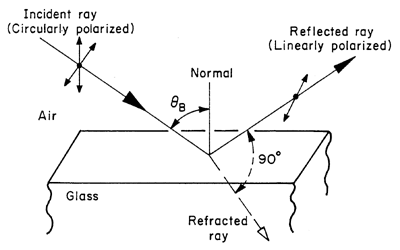Figure 4. Light incident at the Brewster angle, θB, on glass is reflected with complete linear polarization and refracted with a mixture of two perpendicular components.

The Brewster angle is related to the index of refraction n of the material byFor glass, n = 1.5 and θB = 56.3° and for water, n = 1.33 and θB = 53°.

Want to try activities highlighting these principles? Click on the name of this activity to find an easy, hands-on experiment: